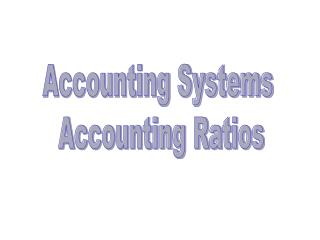DownloadDownload PresentationAccounting Systems Accounting Ratios

# Accounting Systems Accounting Ratios

Télécharger la présentation## Accounting Systems Accounting Ratios

- - - - - - - - - - - - - - - - - - - - - - - - - - - E N D - - - - - - - - - - - - - - - - - - - - - - - - - - -
##### Presentation Transcript

1. Accounting Systems Accounting Ratios

2. Parts of a Financial Statement • Statement of Income • Balance Sheet • Statement of Cash Flow • Statement of Stockholders’ Equity

3. Basic Accounting Identity • Assets = Liabilities + Shareholder’s Equity A = L + Sh Eq

4. 4 Basic Types of Analysis • Operating or activity analysis • Liquidity analysis • Long Term and solvency analysis • Profitability analysis

5. Operating or activity analysis • Inventory a) Turnover • b) Days’ sales in Inventory Cost of goods sold Inventory 365 days Inventory Turnover Sales Accounts Receivable • 2. Receivables a) Turnover • b) Collection Period • Payables a) Turnover • b) Payables Period • Cash Conversion Cycle = Inventory period + • Collection Period - • Payables Period • Assets Turnover 365 days Receivables Turnover Cost of goods sold Payables 365 days Payables Turnover Sales Assets

6. Liquidity Ratios Current Assets Current Liabilities • Current ratio • Quick Ratio • Cash Ratio • Net Working Capital to Assets • Interval measure Current Assets - Inventory Current Liabilities Cash Current Liabilities Net working Capital Total assets Current Assets Average Daily Operating Costs

7. Long term and solvency analysis Liabilities Total Assets • Total Debt Ratios a) Total Debt • b) Debt/ Equity • c) Equity Multiplier Liabilities Total Equity Total Assets Total Equity Earnings before Interest and Taxes (EBIT) Interest • Times Interest Earned • Cash Coverage EBIT + Depreciation Interest

8. Profitability Measures • Profit Margin • Return on Assets • Return on Equity (ROE) • 4. Dupont Identity Net Income Sales Net Income Assets Net Income Equity EBIT X EBI X Net Income X Sales X Assets = EBIT EBI Net Income Sales Assets Equity Equity Tax Interest Net Margin Asset Leverage Return Burden Burden Turnover on Assets

9. Working capital – ST Assets – ST Liabilities Working capital – 1,282 – 672 = 610

10. Operating or activity analysis 1,679 or 872 1.93 • Inventory a) Turnover • b) Days’ sales in Inventory 365 days or 1.93 189 days 2,289 or 345 • 2. Receivables a) Turnover • b) Collection Period • Payables Turnover • Assets Turnover 6.63 55 days 365 days or 6.63 1,679 or 422 3.97 2,289 or 5,589 2.44

11. Liquidity Ratios 1,282 672 • Current ratio • Quick Ratio • Cash Ratio • Net Working Capital to Assets • Interval measure 1.91 445 672 .67 100 672 .14 .11 610 5,589 1,282 6.09 210

12. Long term and solvency analysis .51 2,855 5,589 • Total Debt Ratios a) Total Debt • b) Debt/ Equity • c) Equity Multiplier 1.04 2,855 2,734 5,589 2,734 2.04 Earnings before Interest and Taxes (EBIT) Interest • Times Interest Earned • Cash Coverage EBIT + Depreciation Interest

13. Profitability Measures • Profit Margin • Return on Assets • Return on Equity (ROE) • 4. Dupont Identity 63 2,289 .03 63 5589 .01 63 2734 .02 EBIT X EBI X Net Income X Sales X Assets = EBIT EBI Net Income Sales Assets Equity Equity Tax Interest Net Margin Asset Leverage Return Burden Burden Turnover on Assets